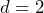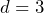# J. Hong: An upper bound for pc in range-R bond percolation in two and three dimensions

Abstract: An upper bound for the critical probability of long range bond percolation inandis obtained by connecting the bond percolation with the SIR epidemic model, thus complementing the lower bound result in Frei and Perkins (2016). A key ingredient is that we establish a uniform bound for the local times of branching random walk by calculating their exponential moments and by using the discrete versions of Tanaka’s formula and Garsia’s Lemma.

This talk is based on the preprint at https://arxiv.org/abs/2107.14173.

## Video